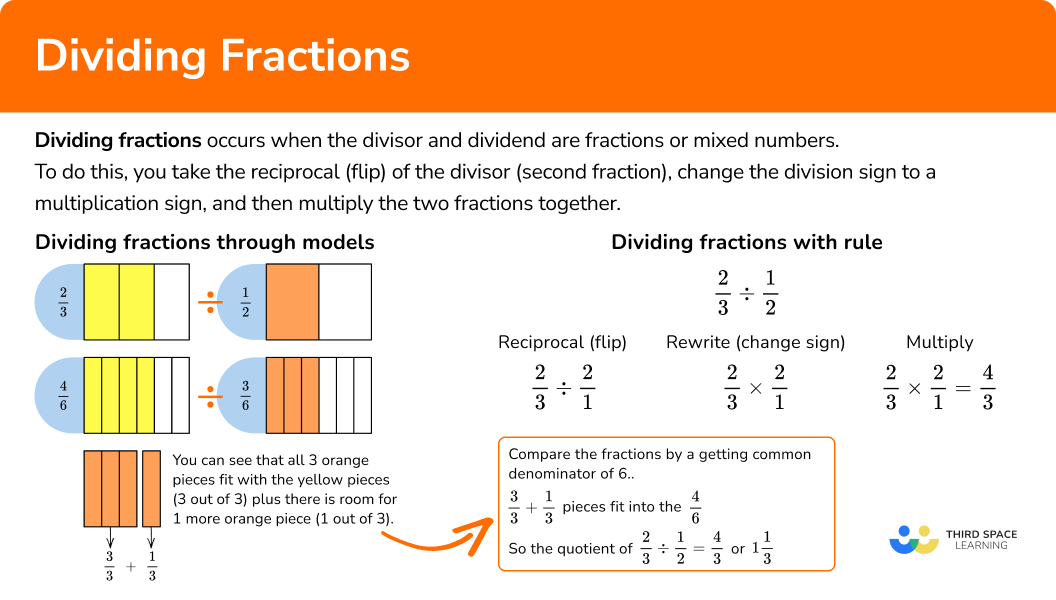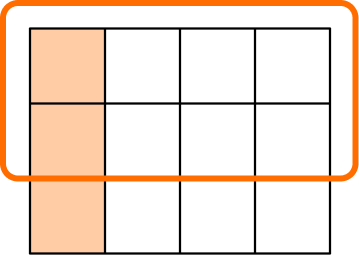# Dividing fractions

Here you’ll learn about dividing fractions including how to divide two fractions, divide fractions by whole numbers, and divide mixed numbers.

Students will first learn about dividing unit fractions in 5th grade math as part of their work in number and operations. They will build on this knowledge and divide all types of fraction in 6th grade math as a part of their work in the number system.

## What is dividing fractions?

Dividing fractions occurs when the divisor and/or dividend are fractions or mixed numbers. The concept of dividing fractions is like dividing whole numbers — you are dividing the number into equal shares.

Let’s look at visual representations of dividing by fractions.

4 circles divided into two equal groups. Results in two circles per group.

2 circles divided into two equal groups. Results in one circle per group.

1 circles divided into two equal groups. Results in \cfrac{1}{2} \, of circle per group.

Do you see a pattern between dividing by 2 and multiplying by \cfrac{1}{2}? They give the same answer.

Dividing by \bf{2} Multiplying by \bf{\frac{1}{2}}
Example \bf{1} 4 \div 2=2 4 \times \cfrac{1}{2} = 2
Example \bf{2} 2 \div 2=1 2 \times \cfrac{1}{2} = 1
Example \bf{3} 1 \div 2= \cfrac{1}{2} 1 \times \cfrac{1}{2} = \cfrac{1}{2}

Let’s look at another visual model.

\cfrac{2}{3} \div \cfrac{1}{2}

in other words, how many \cfrac{1}{2} ’s fit in \cfrac{2}{3}?

This will be easier to see if you compare the fractions by finding a common denominator by using equivalent fractions.

\cfrac{3}{3}+\cfrac{1}{3}=\cfrac{4}{3} pieces fit into the \cfrac{4}{6}

So,

\cfrac{2}{3} \div \cfrac{1}{2}= \cfrac{4}{3} \, or \, 1\cfrac{1}{3}

We can also apply the rule:

KEEP the first fraction, FLIP the second fraction, CHANGE to multiplication.

\cfrac{2}{3}\div \cfrac{1}{2}

Reciprocal (flip second fraction) \; \cfrac{1}{2} → \cfrac{2}{1}

Rewrite to change operation: \; → \cfrac{2}{3}\times \cfrac{2}{1}

Multiply: → \cfrac{2}{3}\times \cfrac{2}{1} =\cfrac{4}{3}

Convert back into mixed number → \cfrac{4}{3} = 1\cfrac{1}{3}

### What is dividing fractions?## Common Core State Standards

How does this relate to 5th grade math and 6th grade math?

• Grade 5 – Number & Operations – Fractions (5.NF.B)
Apply and extend previous understandings of multiplication and division to multiply and divide fractions.

• Grade 6 – Number System (6.NS.A)
Apply and extend previous understandings of multiplication and division to divide fractions by fractions.

## How to divide fractions

In order to divide fractions:

1. Take the reciprocal (flip) of the divisor (second fraction).
2. Change the division sign to a multiplication sign.
3. Multiply the fractions together.
4. If possible, simplify or convert to a mixed number.

In order to divide mixed numbers:

1. Change the mixed number to an improper fraction.
2. Take the reciprocal (flip) of the divisor (second fraction).
3. Change the division sign to a multiplication sign.
4. Multiply the fractions together.
5. If possible, simplify or convert to a mixed number.

In order to solve dividing fraction word problems:

1. Create an equation to model the problem
2. Change any mixed-numbers to an improper fraction.
3. Take the reciprocal (flip) of the divisor (second fractions).
4. Change the division sign to a multiplication sign.
5. Multiply the fractions together.
6. If possible, simplify or convert to a mixed number.

## Dividing fractions examples

### Example 1: dividing a fraction by a fraction

Divide the numbers \, \cfrac{1}{2} \div \cfrac{1}{3}.

1. Take the reciprocal (flip) of the divisor (second fraction).

\cfrac{1}{3} → \cfrac{3}{1}

2Change the division sign to a multiplication sign.

\cfrac{1}{2} \times \cfrac{3}{1}

3Multiply the fractions together.

\cfrac{1}{2} \times \cfrac{3}{1}=\cfrac{3}{2}

4If possible, simplify or convert to a mixed number.

\cfrac{3}{2}=1 \cfrac{1}{2}.

The visual models show the fractions represented with a common denominator. You use equivalent fractions to get the common denominator.

You can see the amount of \cfrac{1}{3} ’s that fit into \cfrac{1}{2}.

\cfrac{2}{2} + \cfrac{1}{2}=\cfrac{3}{2} \; fit or \; 1\cfrac{1}{2}

### Example 2: dividing a fraction by a whole number

Divide the numbers \, \cfrac{1}{2} \div 4.

\cfrac{4}{1} → \cfrac{1}{4}

\cfrac{1}{2} \times \cfrac{1}{4}

\cfrac{1}{2} \times \cfrac{1}{4}=\cfrac{1}{8}

\cfrac{1}{8} is in its simplest form and cannot be changed into a mixed number.

### Example 3: dividing improper fractions

Divide the numbers \, \cfrac{8}{5} \div \cfrac{4}{3}.

\cfrac{4}{3} → \cfrac{3}{4}

\cfrac{8}{5} \times \cfrac{3}{4}

\cfrac{8}{5} \times \cfrac{3}{4}=\cfrac{24}{20}

The common factor between the numerator and denominator is 4. Using equivalent fractions, the numerator and denominator can both be divided by 4.

\cfrac{24 \, \div \, 4}{20 \, \div \, 4}=\cfrac{6}{5}

\cfrac{6}{5} is in simplest form or it can changed into a mixed number 1\cfrac{1}{5}.

### Example 4: dividing a mixed number by a mixed number

Divide the numbers \, 1 \cfrac{1}{2} \div 2 \cfrac{1}{4}.

1 \cfrac{1}{2} → \cfrac{3}{2} \; and \; 2 \cfrac{1}{4} → \cfrac{9}{4}

\cfrac{9}{4} → \cfrac{4}{9}

\cfrac{3}{2} \times \cfrac{4}{9}

\cfrac{3}{2} \times \cfrac{4}{9}=\cfrac{12}{18}

The common factor of numerator and denominator is 6. Using equivalent fractions, the numerator and denominator can both be divided by 6.

\cfrac{12 \, \div \, 6}{18 \, \div \, 6} = \cfrac{2}{3}

\cfrac{2}{3} is in lowest terms.

### Example 5: word problem dividing with a whole number

There are 12 pizzas eaten at a party. Each person ate a quarter of the pizza. How many people ate pizza?

12\div\cfrac{1}{4}= \, ? \;

No mixed numbers.

\cfrac{1}{4} → \cfrac{4}{1}

\cfrac{12}{1} \div \cfrac{4}{1}

\cfrac{12}{1} \div \cfrac{4}{1}=48

No simplifying needed

48 people

Below is a visual model showing 12 pizzas divided into quarters. There are 48 quarters, which means 48 people were at the party.

### Example 6: word problem dividing mixed numbers

If it requires \cfrac{1}{16} of a pound of beef to make a hamburger patty, how many patties can be made 1\cfrac{1}{8} with pounds of beef?

1\cfrac{1}{8} \div \cfrac{1}{16}= \, ?

1\cfrac{1}{8} → \cfrac{9}{8}

\cfrac{1}{16} → \cfrac{16}{1}

\cfrac{9}{8} \times \cfrac{16}{1}

\cfrac{9}{8} \times \cfrac{16}{1}=\cfrac{144}{8}

\cfrac{144}{8} has a common factor of 8

\cfrac{144 \, \div \, 8}{8 \, \div \,8}= \cfrac{18}{1}=18

18 beef patties

### Teaching tips for dividing fractions

• Division of fractions is challenging for students to formulate understanding. Utilize hands-on visual models and/or digital models so that students can develop understanding.

• Explore patterns so that students can make sense of the steps involved in dividing fractions

• Although practicing dividing by fractions is important, do not rely on practice worksheets to develop deep understanding. Using visual models, hands-on activities, and digital games are very effective alternatives to multiplication fractions worksheets.

• Although conceptual understanding is essential to learning division of fractions, the simple steps of keep – change – flip help struggling students remember.

### Our favorite mistakes

• Taking the reciprocal of the dividend (first fraction) instead of the divisor (second fraction)
• Not changing the operation to multiplication
• Taking the reciprocal of both the dividend and the divisor
• After taking the reciprocal of the divisor, cross multiplying the fractions instead of multiplying the fractions

### Practice dividing fractions questions

1. Divide the fractions.

\cfrac{2}{7} \div \cfrac{3}{5}

\cfrac{21}{10}\cfrac{10}{21}\cfrac{6}{35}\cfrac{35}{6}Keep the first fraction \; \cfrac{2}{7}

Take the reciprocal (flip) the second fraction \; \cfrac{3}{5} → \cfrac{5}{3}

Change division to multiplication \; \cfrac{2}{7}\times \cfrac{5}{3}

Multiply \; \cfrac{2}{7}\times \cfrac{5}{3}= \cfrac{10}{21}

\cfrac{10}{21} \; is in lowest terms.

2. Divide the numbers.

\cfrac{6}{7}\div \cfrac{10}{3}

\cfrac{60}{21}\cfrac{35}{9}\cfrac{9}{35}\cfrac{6}{21}\cfrac{6}{7}\div \cfrac{10}{3}

Keep the first fraction \; \cfrac{6}{7}

Take the reciprocal (flip) the second fraction \; \cfrac{10}{3} → \cfrac{3}{10}

Change division to multiplication \; \cfrac{6}{7}\times \cfrac{3}{10}

Multiply the fractions \; \cfrac{6}{7}\times \cfrac{3}{10}= \cfrac{18}{70}

\cfrac{18}{70} \; has a common factor of 2. Use equivalent fractions to simplify.

\cfrac{18 \, \div \, 2}{70 \, \div \, 2}= \cfrac{9}{35}

\cfrac{9}{35} \; is in lowest terms.

3. Divide the numbers.

\cfrac{4}{9}\div 8

\cfrac{32}{9}3\cfrac{5}{9}\cfrac{4}{17}\cfrac{1}{18}Keep the first dividend (first fraction) \; \cfrac{4}{9}

Take the reciprocal (flip) the divisor (second fraction) \; 8 → \cfrac{1}{8}

Change division to multiplication \; \cfrac{4}{9}\times \cfrac{1}{8}

Multiply the fractions \; \cfrac{4}{9}\times \cfrac{1}{8}= \cfrac{4}{72}

\cfrac{4}{72} \; has a common factor of 4. Use equivalent fractions to simplify.

\cfrac{4 \, \div \, 4}{72 \, \div \, 4}=\cfrac{1}{18} \; is in lowest terms.

4. Divide the numbers.

2\cfrac{3}{4}\div 1\cfrac{2}{3}

1\cfrac{13}{20}4\cfrac{7}{12}\cfrac{55}{12}2\cfrac{2}{7}Change the mixed numbers to improper fractions

2\cfrac{3}{4} → \cfrac{11}{4}

1\cfrac{2}{3} → \cfrac{5}{3}

Keep the dividend (first fraction) \; \cfrac{11}{4}

Take the reciprocal (flip) the divisor (second fraction) \; \cfrac{5}{3} → \cfrac{3}{5}

Change to multiplication \; \cfrac{11}{4}\times \cfrac{3}{5}

Multiply the fractions \; \cfrac{11}{4}\times \cfrac{3}{5}=\cfrac{33}{20}

Change back into a mixed number \cfrac{33}{20} → 1\cfrac{13}{20}

5. Annie bought three dolls to give to her nieces. If she uses \cfrac{7}{8} of a roll of wrapping paper to wrap the dolls. What fraction of the gift wrap did she use to package each doll?

3\cfrac{3}{7} of the wrap1\cfrac{4}{7} of the wrap\cfrac{7}{11} of the wrap\cfrac{7}{24} of the wrapCreate the equation \; \cfrac{7}{8}\div {3}= \, ?

Find the reciprocal (flip) of the divisor (second fraction) \; 3 → \cfrac{1}{3}

Change to multiplication \; \cfrac{7}{8}\times \cfrac{1}{3}

Multiply the fractions \; \cfrac{7}{8}\times \cfrac{1}{3}= \cfrac{7}{24}

\cfrac{7}{24} is in lowest terms.

\cfrac{7}{24} of the gift wrap was used for each package.

6. How many pieces of rope measuring \cfrac{3}{4} of a foot can be cut from a rope that is 4\cfrac{1}{8} feet in length?

5\cfrac{1}{2} pieces of rope5\cfrac{1}{8} pieces of rope3\cfrac{1}{8} pieces of rope3 pieces of ropeCreate the equation \; 4\cfrac{1}{8}\div \cfrac{3}{4}= \, ?

Convert mixed number to improper fractions \; 4\cfrac{1}{8} → \cfrac{33}{8}

Take reciprocal (flip) of the second fraction \; \cfrac{3}{4} → \cfrac{4}{3}

Change to multiplication \; \cfrac{33}{8}\times \cfrac{4}{3}

Multiply together \; \cfrac{33}{8}\times \cfrac{4}{3}=\cfrac{132}{24}

Convert to a mixed number 5\cfrac{12}{24}

The numerator and denominator have a common factor of 12. Using equivalent fractions,

\cfrac{12 \, \div \, 12}{24 \, \div \, 12}=\cfrac{1}{2}

5\cfrac{12}{24}=5\cfrac{1}{2}

5\cfrac{1}{2} pieces of rope

## Dividing fractions FAQs:

Is dividing fractions the same as multiplying fractions?

No, when you divide fractions, you multiply by the reciprocal of the divisor. When you multiply fractions, you simply multiply the fractions together and do not take the reciprocal of the second fraction.

With division of fractions, do you have to get a common denominator?

No, you do not have to get a common denominator to divide fractions. However, getting a common denominator can be useful when using visual models for the division of fractions.

What other visual models can be used for dividing fractions?

You can use a grid model to divide fractions where the number of columns is the denominator of the dividend and the number of rows is the denominator of the divisor. You shade the number of columns represented by the numerator of the dividend and circle the number of rows represented by the numerator of the divisor. The quotient is the total number of shaded squares over the total number of circled squares.

\cfrac{1}{4} \div \cfrac{2}{3} = \cfrac{3}{8}## Still stuck?

At Third Space Learning, we specialize in helping teachers and school leaders to provide personalized math support for more of their students through high-quality, online one-on-one math tutoring delivered by subject experts.

Each week, our tutors support thousands of students who are at risk of not meeting their grade-level expectations, and help accelerate their progress and boost their confidence.